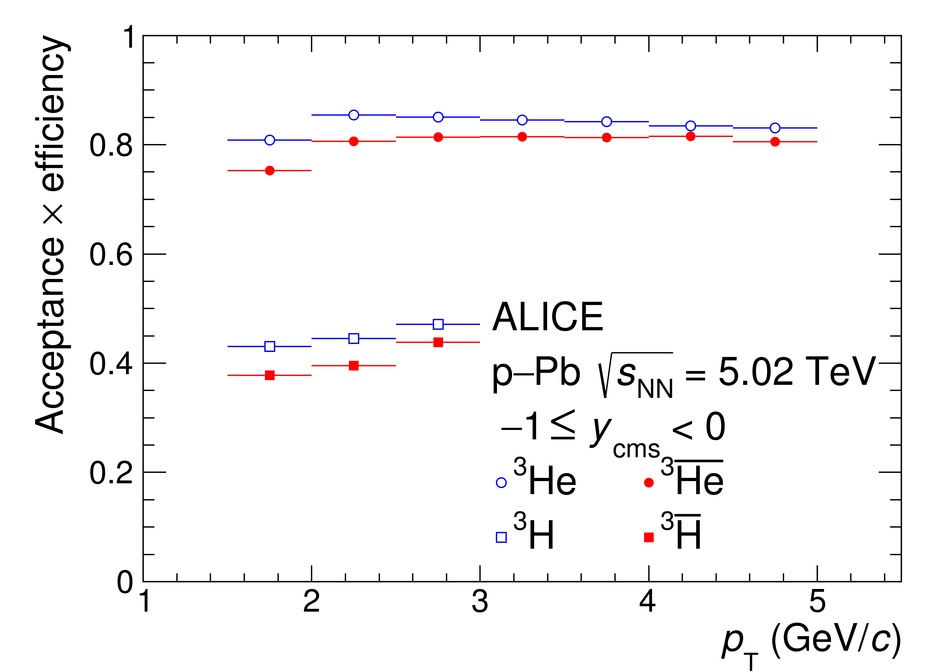# Figure 3

 The acceptance $\times$ efficiency as a function of \pt is shown for $^3\overline{\mathrm{He}}$ and $^3\text{He}$ as well as for $^3\overline{\mathrm{H}}$ and $^3$H.